# MARKET EQUILIBRIUM, DISEQUILIBRIUM AND EFFECT OF CHANGES IN DEMAND AND SUPPLY

0

The relationship between market demand and supply is aimed at determining the equilibrium in the market.

The equilibrium is the point where the upward-sloping supply curve intersects the downward-sloping demand curve.

This means the price and quantity of the sellers are the same as that of the buyer.

Simply said, equilibrium is a situation in which the quantity and price offered by the sellers are the same as the quantity and price of the buyers

The price where this occurs is called the equilibrium price or market clearing price, and the quantity where it occurs is called the equilibrium quantity or market-clearing quantity

#### Example

The monthly demand and supply curve for shoes is illustrated below.

Price (€)Quantity suppliedQuantity demanded
80010007000
100020006000
120030005000
140040004000
160050003000
180060002000

As can be seen, the equilibrium price is €1400  and the equilibrium quantity is 4000.

At the price of €1400, sellers were willing to sell 4000 shoes, which is the same quantity that the buyers want.

Therefore, there will be no excess demand or supply at €1400. This means that there is no 'leftover', and the market has cleared.

As you will see below, we can visualize this on a graph.

## Disequilibrium

Disequilibrium occurs when the quantity demanded does not always equal the quantity supplied.

It occurs when the price charged is above or below the equilibrium price.

There will be excess supply if the price is set above the equilibrium price.

There will be excess demand if it is charged below the equilibrium price

### Excess supply(or surplus)

This is a situation where the quantity supplied exceeds the quantity demanded at a prevailing price. This will result in a surplus.

Excess supply is usually associated with high prices. High prices will lead to a diminished quantity demanded and an enhanced quantity supplied.

Why enhanced quantity supplied?

The law of supply states that "the higher the price, the higher the quantity supplied and vice versa. Hence, we would have an enhanced quantity supplied".

If you are not familiar with supply, see my blog post on supply

Why diminished the quantity demanded?

The law of demand states that "the higher the price, the lower the quantity demanded and vice versa. Hence, we would have a diminished quantity demanded".

If you are not yet familiar with demand, refer to this post here

Going by our earlier example, when the price was €1600, the quantity of shoes demanded is 3000 and the quantity supplied is 5000. This signifies that sellers are willing to sell 2000 more shoes than consumers are willing to buy.

This would cause a surplus in the market.

But, if the seller wants to sell all his shoes, what will he do?

He will have to reduce the price of his shoes so that it attracts buyers. This price reduction would lead to a higher quantity demanded.

However, the suppliers can not produce much(due to the low prices).

Eventually, the market will be balanced as price and quantity would move back to their equilibrium.

So, excess supply will induce sellers into reducing prices so that supply matches the available demand, resulting in market equilibrium.

### Excess demand (or shortage)

This is a situation where quantity demanded exceeds quantity supplied at prevailing prices, thereby, leading to a shortage. Excess supply is usually correlated with low prices.

Now, think of what would happen if the price was €1200. The number of shoes demanded(5000) is 2000 more than the number of shoes supplied(3000).

In this case, buyers want more than 2000 more shoes than the quantity offered by suppliers.

Suppliers, knowing that there is a shortage, would use the opportunity to increase their profit margin by selling at higher prices.

However, as the price rises, the quantity demanded will fall (law of demand). Once again, the market will adjust to its equilibrium point.

Note, that this process of adjustment does not always happen instantly, Some may take a very long time.

The point is that economic pressures will always cause the market to move back to its equilibrium quantity and price.

## Changes In Equilibrium

So far, we have been looking at the effect of price changes on the market quantity.

However, a price change will not shift the equilibrium point, it will only cause excess demand and excess supply.

So, what causes the equilibrium to shift?

Non-price Factors!!!

Like I told you before, a change in non-price factors will cause an entire shift in the positions of the demand and supply curve.

A shift in the position of the demand and supply curve will mean that the equilibrium point would move to a new point.

For example, if schools were shut down for months, many students may not buy a pen, as illustrated in our new schedule.

Price (€)Quantity suppliedQuantity demandedNew quantity demanded
800100070005000
1000200060004000
1200300050003000
1400400040002000
1600500030001000
180060002000500

As you can see, the equilibrium price and quantity( which initially were,€1600 and 4000 respectively) are re-established as €1200 and 3000 respectively.

Related post

### Impacts of changes in demand and supply on the equilibrium

There are eight causes of equilibrium price and quantity changes which are:

1. Increase in demand while supply remains constant: When demand increases, the equilibrium price and quantity will also increase. The accompanying diagram illustrates this: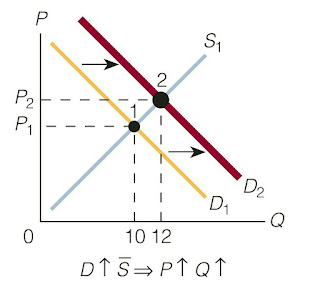Image credit: Roger.A. Arnold's microeconomics

2. Decrease in demand while price remains constant: When demand decrease, both the equilibrium price and quantity will decrease. Here is a graphical representation.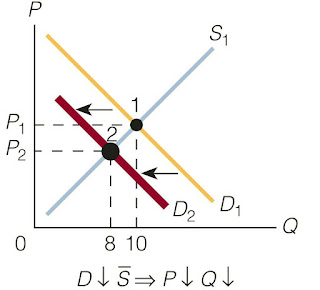Image credit: Roger.A. Arnold's microeconomics

3. Increase in supply while demand is constant: When supply increase, the equilibrium price will decrease while the equilibrium quantity will increase as shown below: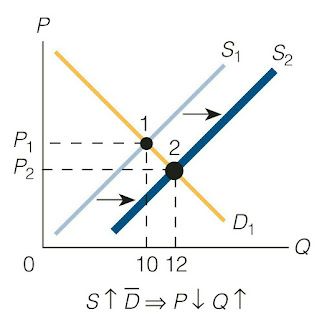Image credit: Roger.A. Arnold's microeconomics
4. Decrease in supply while demand is constant: When supply decrease, the equilibrium price increase, and the equilibrium quantity decrease.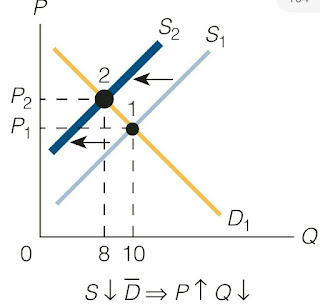Image credit: Roger.A. Arnold's microeconomics
5. Demand rises and supply falls by an equal amount: when demand rises and supply fall by the same amount, the equilibrium price rises while the equilibrium quantity stays the same.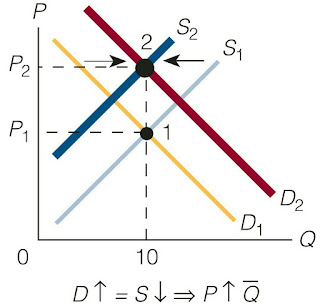Image credit: Roger.A. Arnold's microeconomics
6. Demand falls and supply rises by an equal amount: When demand falls and supply rises by the same amount, the equilibrium price falls while the equilibrium quantity remains constant.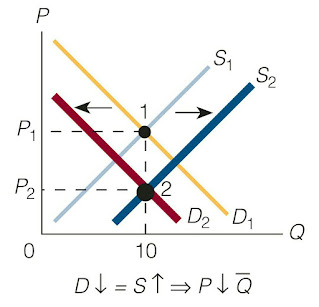Image credit: Roger.A. Arnold's microeconomics
7. Demand rises by a greater amount than supply falls: when demand increases by a larger amount than supply decreases, both equilibrium price and quantity rise as shown in the accompanying graph.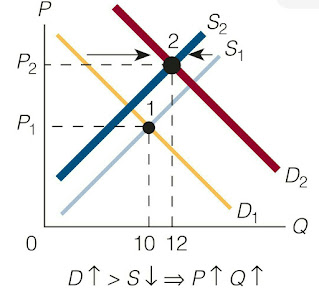Image credit: Roger.A. Arnold's microeconomics
8. Demand rises by a smaller amount than supply falls: When demand rises by a lesser amount than supply falls, the equilibrium price rises while the equilibrium quantity falls.
Before we conclude, here are some other things you should note:

1. When the increase in demand is larger than the decrease in supply, equilibrium price and quantity will rise.

2. If demand increases by a larger amount than supply increases, equilibrium price and quantity will rise.

3. If demand and supply decrease by the same amount, the equilibrium quantity falls, while the equilibrium price stays the same.

4. If demand and supply increase by the same amount, the equilibrium quantity rises while the equilibrium price stays the same.

In this next post, you will practice some examples that will solidify your knowledge of equilibrium.

In the meantime, if you have got questions, do well to ask our telegram community.
Tags